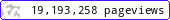•••••MadAsMaths.com :: I.Y.G.B. Practice Papers :: Undergraduate Methods Papers :: Mathematical Methods 3 Practice Papers

I.Y.G.B. MATHEMATICAL METHODS 3 PRACTICE PAPERS

These I.Y.G.B. practice papers follow the most common syllabi for a second year degree in Mathematics, Engineering or Science.

The examined topics are listed at the front cover of each paper.

All papers have model solutions.

 mathmeth3_a.pdfmathmeth3_a_solutions.pdfmathmeth3_b.pdfmathmeth3_b_solutions.pdfmathmeth3_c.pdfmathmeth3_c_solutions.pdfmathmeth3_d.pdfmathmeth3_d_solutions.pdfmathmeth3_e.pdfmathmeth3_e_solutions.pdfmathmeth3_f.pdfmathmeth3_f_solutions.pdfmathmeth3_g.pdfmathmeth3_g_solutions.pdfmathmeth3_h.pdfmathmeth3_h_solutions.pdfmathmeth3_i.pdfmathmeth3_i_solutions.pdfmathmeth3_j.pdfmathmeth3_j_solutions.pdfmathmeth3_k.pdfmathmeth3_k_solutions.pdfmathmeth3_l.pdfmathmeth3_l_solutions.pdfmathmeth3_m.pdfmathmeth3_m_solutions.pdfmathmeth3_n.pdfmathmeth3_n_solutions.pdfmathmeth3_o.pdfmathmeth3_o_solutions.pdfmathmeth3_p.pdfmathmeth3_p_solutions.pdfmathmeth3_q.pdfmathmeth3_q_solutions.pdfmathmeth3_r.pdfmathmeth3_r_solutions.pdfmathmeth3_s.pdfmathmeth3_s_solutions.pdfmathmeth3_t.pdfmathmeth3_t_solutions.pdfmathmeth3_u.pdfmathmeth3_u_solutions.pdfmathmeth3_v.pdfmathmeth3_v_solutions.pdfmathmeth3_w.pdfmathmeth3_w_solutions.pdfmathmeth3_x.pdfmathmeth3_x_solutions.pdfmathmeth3_y.pdfmathmeth3_y_solutions.pdfmathmeth3_z.pdfmathmeth3_z_solutions.pdf